GeeksforGeeks App
Open AppBrowser
Continue

# How to drop one or multiple columns in Pandas Dataframe

Let’s discuss how to drop one or multiple columns in Pandas Dataframe. To Delete a column from a Pandas DataFrame or Drop one or more than one column from a DataFrame can be achieved in multiple ways.

Create a simple Dataframe with dictionary of lists, say column names are A, B, C, D, E. In this article, we will cover 6 different methods to delete some columns from Pandas DataFrame.

## Python3

 `# Import pandas package``import` `pandas as pd`` ` `# create a dictionary with five fields each``data ``=` `{``    ``'A'``: [``'A1'``, ``'A2'``, ``'A3'``, ``'A4'``, ``'A5'``],``    ``'B'``: [``'B1'``, ``'B2'``, ``'B3'``, ``'B4'``, ``'B5'``],``    ``'C'``: [``'C1'``, ``'C2'``, ``'C3'``, ``'C4'``, ``'C5'``],``    ``'D'``: [``'D1'``, ``'D2'``, ``'D3'``, ``'D4'``, ``'D5'``],``    ``'E'``: [``'E1'``, ``'E2'``, ``'E3'``, ``'E4'``, ``'E5'``]}`` ` `# Convert the dictionary into DataFrame``df ``=` `pd.DataFrame(data)`` ` `df`

Output: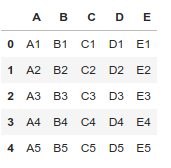### Method 1: Drop Columns from a Dataframe using drop() method.

Example 1: Remove specific single columns.

## Python3

 `# Import pandas package``import` `pandas as pd`` ` `# create a dictionary with five fields each``data ``=` `{``    ``'A'``: [``'A1'``, ``'A2'``, ``'A3'``, ``'A4'``, ``'A5'``],``    ``'B'``: [``'B1'``, ``'B2'``, ``'B3'``, ``'B4'``, ``'B5'``],``    ``'C'``: [``'C1'``, ``'C2'``, ``'C3'``, ``'C4'``, ``'C5'``],``    ``'D'``: [``'D1'``, ``'D2'``, ``'D3'``, ``'D4'``, ``'D5'``],``    ``'E'``: [``'E1'``, ``'E2'``, ``'E3'``, ``'E4'``, ``'E5'``]}`` ` `# Convert the dictionary into DataFrame``df ``=` `pd.DataFrame(data)`` ` `# Remove column name 'A'``df.drop([``'A'``], axis``=``1``)`

Output: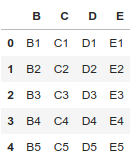Example 2: Remove specific multiple columns.

## Python3

 `# Import pandas package``import` `pandas as pd`` ` `# create a dictionary with five fields each``data ``=` `{``    ``'A'``: [``'A1'``, ``'A2'``, ``'A3'``, ``'A4'``, ``'A5'``],``    ``'B'``: [``'B1'``, ``'B2'``, ``'B3'``, ``'B4'``, ``'B5'``],``    ``'C'``: [``'C1'``, ``'C2'``, ``'C3'``, ``'C4'``, ``'C5'``],``    ``'D'``: [``'D1'``, ``'D2'``, ``'D3'``, ``'D4'``, ``'D5'``],``    ``'E'``: [``'E1'``, ``'E2'``, ``'E3'``, ``'E4'``, ``'E5'``]}`` ` `# Convert the dictionary into DataFrame``df ``=` `pd.DataFrame(data)`` ` `# Remove two columns name is 'C' and 'D'``df.drop([``'C'``, ``'D'``], axis``=``1``)`` ` `# df.drop(columns =['C', 'D'])`

Output: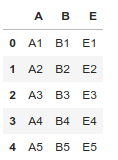Example 3: Remove columns based on column index.

## Python3

 `# Import pandas package``import` `pandas as pd`` ` `# create a dictionary with five fields each``data ``=` `{``    ``'A'``: [``'A1'``, ``'A2'``, ``'A3'``, ``'A4'``, ``'A5'``],``    ``'B'``: [``'B1'``, ``'B2'``, ``'B3'``, ``'B4'``, ``'B5'``],``    ``'C'``: [``'C1'``, ``'C2'``, ``'C3'``, ``'C4'``, ``'C5'``],``    ``'D'``: [``'D1'``, ``'D2'``, ``'D3'``, ``'D4'``, ``'D5'``],``    ``'E'``: [``'E1'``, ``'E2'``, ``'E3'``, ``'E4'``, ``'E5'``]}`` ` `# Convert the dictionary into DataFrame``df ``=` `pd.DataFrame(data)`` ` `# Remove three columns as index base``df.drop(df.columns[[``0``, ``4``, ``2``]], axis``=``1``, inplace``=``True``)`` ` `df`

Output: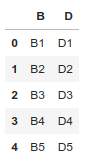### Method 2: Drop Columns from a Dataframe using iloc[] and drop() method.

Remove all columns between a specific column to another column.

## Python3

 `# Import pandas package``import` `pandas as pd``# create a dictionary with five fields each``data ``=` `{``    ``'A'``: [``'A1'``, ``'A2'``, ``'A3'``, ``'A4'``, ``'A5'``],``    ``'B'``: [``'B1'``, ``'B2'``, ``'B3'``, ``'B4'``, ``'B5'``],``    ``'C'``: [``'C1'``, ``'C2'``, ``'C3'``, ``'C4'``, ``'C5'``],``    ``'D'``: [``'D1'``, ``'D2'``, ``'D3'``, ``'D4'``, ``'D5'``],``    ``'E'``: [``'E1'``, ``'E2'``, ``'E3'``, ``'E4'``, ``'E5'``]}`` ` `# Convert the dictionary into DataFrame``df ``=` `pd.DataFrame(data)`` ` `# Remove all columns between column index 1 to 3``df.drop(df.iloc[:, ``1``:``3``], inplace``=``True``, axis``=``1``)`` ` `df`

Output: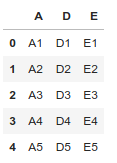### Method 3: Drop Columns from a Dataframe using ix() and drop() method.

Remove all columns between a specific column name to another column’s name.

## Python3

 `# Import pandas package``import` `pandas as pd`` ` `# create a dictionary with five fields each``data ``=` `{``    ``'A'``: [``'A1'``, ``'A2'``, ``'A3'``, ``'A4'``, ``'A5'``],``    ``'B'``: [``'B1'``, ``'B2'``, ``'B3'``, ``'B4'``, ``'B5'``],``    ``'C'``: [``'C1'``, ``'C2'``, ``'C3'``, ``'C4'``, ``'C5'``],``    ``'D'``: [``'D1'``, ``'D2'``, ``'D3'``, ``'D4'``, ``'D5'``],``    ``'E'``: [``'E1'``, ``'E2'``, ``'E3'``, ``'E4'``, ``'E5'``]}`` ` `# Convert the dictionary into DataFrame``df ``=` `pd.DataFrame(data)`` ` `# Remove all columns between column name 'B' to 'D'``df.drop(df.ix[:, ``'B'``:``'D'``].columns, axis``=``1``)`

Output: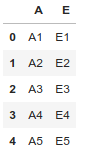### Method 4: Drop Columns from a Dataframe using loc[] and drop() method.

Remove all columns between a specific column name to another column’s name.

## Python3

 `# Import pandas package``import` `pandas as pd`` ` `# create a dictionary with five fields each``data ``=` `{``    ``'A'``: [``'A1'``, ``'A2'``, ``'A3'``, ``'A4'``, ``'A5'``],``    ``'B'``: [``'B1'``, ``'B2'``, ``'B3'``, ``'B4'``, ``'B5'``],``    ``'C'``: [``'C1'``, ``'C2'``, ``'C3'``, ``'C4'``, ``'C5'``],``    ``'D'``: [``'D1'``, ``'D2'``, ``'D3'``, ``'D4'``, ``'D5'``],``    ``'E'``: [``'E1'``, ``'E2'``, ``'E3'``, ``'E4'``, ``'E5'``]}`` ` `# Convert the dictionary into DataFrame``df ``=` `pd.DataFrame(data)`` ` `# Remove all columns between column name 'B' to 'D'``df.drop(df.loc[:, ``'B'``:``'D'``].columns, axis``=``1``)`

Output: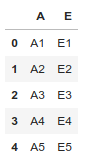Note: Different loc() and iloc() is iloc() exclude last column range element.

### Method 5: Drop Columns from a Dataframe in an iterative way.

Remove all columns between a specific column name to another column’s name.

## Python3

 `# Import pandas package``import` `pandas as pd`` ` `# create a dictionary with five fields each``data ``=` `{``    ``'A'``: [``'A1'``, ``'A2'``, ``'A3'``, ``'A4'``, ``'A5'``],``    ``'B'``: [``'B1'``, ``'B2'``, ``'B3'``, ``'B4'``, ``'B5'``],``    ``'C'``: [``'C1'``, ``'C2'``, ``'C3'``, ``'C4'``, ``'C5'``],``    ``'D'``: [``'D1'``, ``'D2'``, ``'D3'``, ``'D4'``, ``'D5'``],``    ``'E'``: [``'E1'``, ``'E2'``, ``'E3'``, ``'E4'``, ``'E5'``]}`` ` `# Convert the dictionary into DataFrame``df ``=` `pd.DataFrame(data)``for` `col ``in` `df.columns:``    ``if` `'A'` `in` `col:``        ``del` `df[col]`` ` `df`

Output: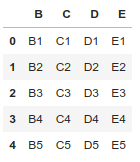## Python3

 `# Import pandas package``import` `pandas as pd`` ` `# create a dictionary with five fields each``data ``=` `{``    ``'A'``: [``'A1'``, ``'A2'``, ``'A3'``, ``'A4'``, ``'A5'``],``    ``'B'``: [``'B1'``, ``'B2'``, ``'B3'``, ``'B4'``, ``'B5'``],``    ``'C'``: [``'C1'``, ``'C2'``, ``'C3'``, ``'C4'``, ``'C5'``],``    ``'D'``: [``'D1'``, ``'D2'``, ``'D3'``, ``'D4'``, ``'D5'``],``    ``'E'``: [``'E1'``, ``'E2'``, ``'E3'``, ``'E4'``, ``'E5'``]}`` ` `# Convert the dictionary into DataFrame``df ``=` `pd.DataFrame(data)``df.pop(``'B'``)`` ` `df`

Output:

```A    C    D    E
0    A1    C1    D1    E1
1    A2    C2    D2    E2
2    A3    C3    D3    E3
3    A4    C4    D4    E4
4    A5    C5    D5    E5```

My Personal Notes arrow_drop_up